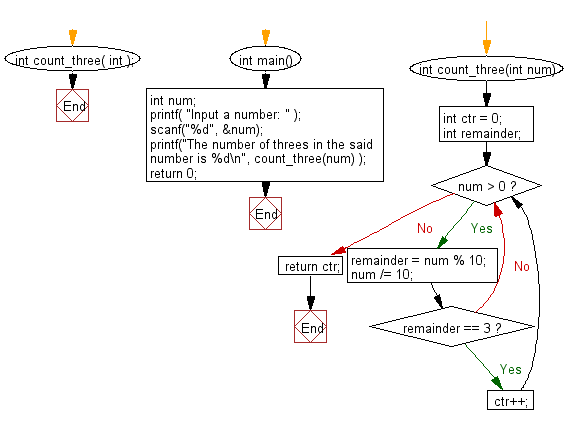﻿ C Program: Count the number of 3s in the given integer

# C Exercises: Reads an integer and count number of 3s in the given number

## C Basic Declarations and Expressions: Exercise-83 with Solution

Write a C program that reads an integer (7 digits or fewer) and counts the number of 3s in the given number.

Sample Input: 538453

Sample Solution:

C Code:

``````#include<stdio.h>

// Function to count the occurrences of digit '3' in a number
int count_three(int);

int main()
{
int num;
// Prompt user for input
printf("Input a number: ");
scanf("%d", &num);
// Call the function count_three and print the result
printf("The number of threes in the said number is %d\n", count_three(num) );
return 0;
}

int count_three(int num)
{
int ctr = 0;    // Initialize counter variable
int remainder;  // Variable to store the remainder

// Loop until num is greater than 0
while(num> 0) {
remainder = num % 10;   // Get the last digit
num /= 10;              // Remove the last digit

if(remainder == 3)      // Check if the last digit is 3
ctr++;              // Increment counter if it is 3
}

return ctr;                 // Return the count of occurrences of 3
}
``````

Sample Output:

```Input a number: The number of threes in the said number is 2.
```

Pictorial Presentation:Flowchart:C programming Code Editor:

What is the difficulty level of this exercise?

Test your Programming skills with w3resource's quiz.

﻿